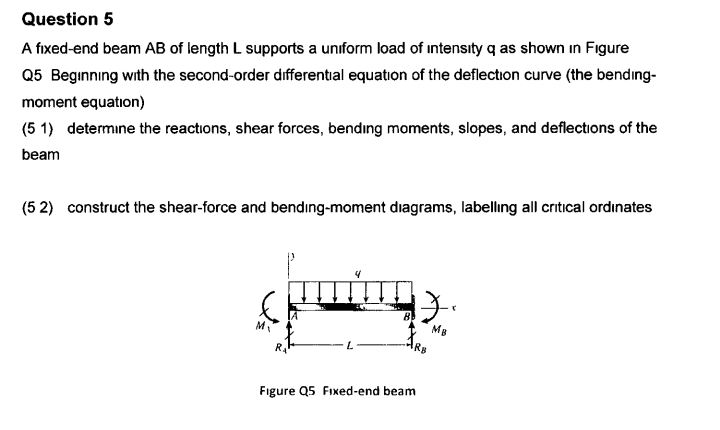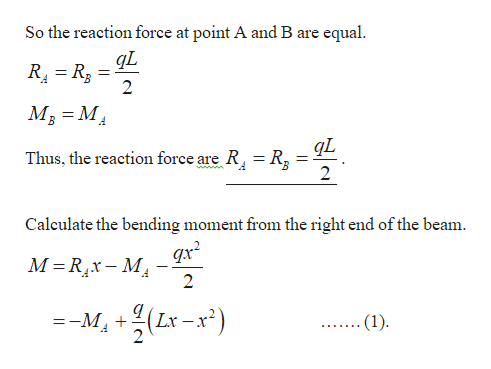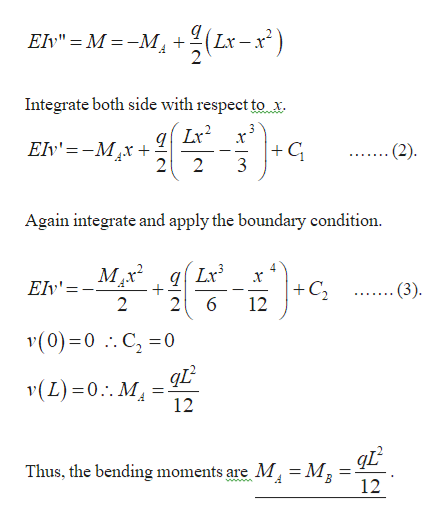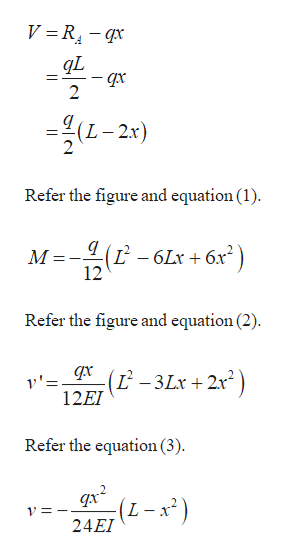Question 5A fixed-end beam AB of length L supports a uniform load of intensity q as shown in FigureQ5 Beginning with the second-order differental equation of the deflection curve (the bendingmoment equation)(5 1) determine the reactions, shear forces, bending moments, slopes, and deflections of thebeamconstruct the shear-force and bending-moment diagrams, labelling all critical ordinates(5 2)MgMvRsLRAFigure Q5 Fixed-end beam

Questionhelp_outlineImage TranscriptioncloseQuestion 5 A fixed-end beam AB of length L supports a uniform load of intensity q as shown in Figure Q5 Beginning with the second-order differental equation of the deflection curve (the bending moment equation) (5 1) determine the reactions, shear forces, bending moments, slopes, and deflections of the beam construct the shear-force and bending-moment diagrams, labelling all critical ordinates (5 2) Mg Mv Rs L RA Figure Q5 Fixed-end beam fullscreen
Step 1

Refer the given figure, the given figure is symmetry and loaded as equilibrium.help_outlineImage TranscriptioncloseSo the reaction force at point A and B are equal gL R R3 2 М, %3 М, Thus, the reaction force are R R3 Calculate the bending moment from the right end of the beam M Rx- M 2 -- М, + (Lx .(1) 2 (ck-x7)7 fullscreen
Step 2

Write the differential equation for the fixed end beam.help_outlineImage TranscriptioncloseLx-x) Elv" = M -M + Integrate both side with respect to x Elv'=-Mx+ 2 C 3 (2) 2 Again integrate and apply the boundary condition. Ev'=-Mx* gLr 2 C 12 (3) + 2 (0) 0 C 0 .. v(L) 0. M 12 Thus, the bending moments are M, = Mg 12 fullscreen
Step 3

Refer the figure, write the ...help_outlineImage TranscriptioncloseV R4- qx 2 (L-2: Refer the figure and equation (1) (L 12 6Lr 6x M Refer the figure and equation (2) 1 -3Lx + 2x) 12EI Refer the equation (3) qx2 (L-x2) 24EI fullscreen

Want to see the full answer?

See Solution

Want to see this answer and more?

Our solutions are written by experts, many with advanced degrees, and available 24/7

See Solution
Tagged in

Solid Mechanics